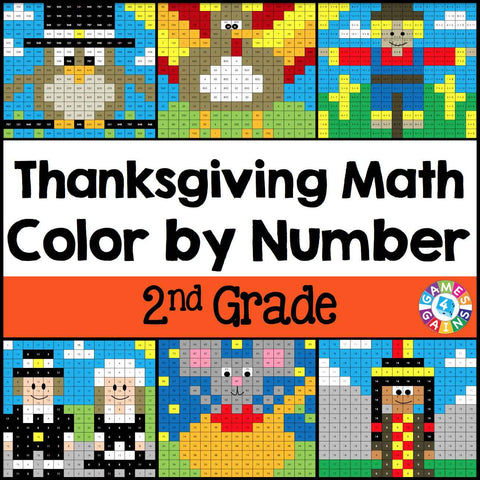Thanksgiving Math Color-by-Number - 2nd Grade

• \$480

Thanksgiving Math Color-by-Number set comes with 6 Thanksgiving math color-by-number activities for reviewing 2nd grade math skills. This Thanksgiving math set is perfect to use in centers, in small groups, or with the whole class!

Included with this Thanksgiving Math Color-by-Number set:
Instructions
6 color by number worksheets
6 student problem worksheets
6 teacher answer sheets

Here are the skills covered by these Thanksgiving math color-by-number activities:

• Determining the value of a digit in a two or three-digit number
• Counting to 1,000
• Finding equivalent addition equations (addition facts to 20)
• Finding the unknown in subtraction problems (subtraction facts to 20)
• Solving two-step addition and subtraction words problems to 20
• Adding and subtracting a two-digit number and a one-digit number

Want to learn more about what you'll get? Check out the preview to see a sample of the actual pages.